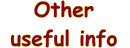Main Other useful info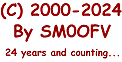Last modified 2020-04-17

## uPD858C

### PLL frequency synthesizer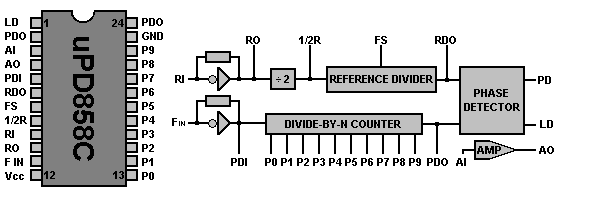### Overview

This PLL-circuit use a 10 bit BCD programmable divide-by-N counter for 399 channels.

### Down-converting of the frequency to the divider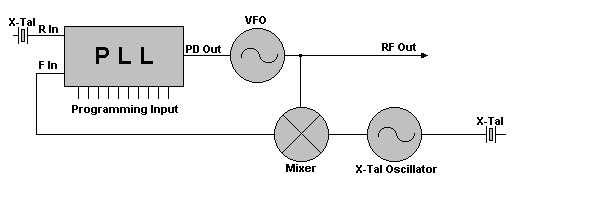This PLL circuit use a mixer and a XTAL oscillator to convert the output frequency f OUT to the f IN to the PLL Circuit.
The XTAL frequency is f XTAL = f OUT - f IN
The output frequency can be changed by changing the mixing-XTAL or add a new mixing-XTAL to the oscillator.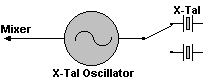Pin Name Description
1 LD Loop detector output - High = Unlocked, Low = Locked
2 PD out Phase Detector output
3 AI Loop filter Amplifier Input
4 AO Loop filter Amplifier Output
5 PDI Programmable Divider Input
6 RDO Reference Divider Output
7 FS Frequency Select input - High=10 KHz, Low=5 KHz
8 1/2R Reference frequency divided by 2
9 RI Reference oscillator Input (XTAL)
10 RO Reference oscillator Output (XTAL)
11 Fin VCO oscillator input
12 VCC Power supply (+5 VDC)
13 P0 Programmable input 0
14 P1 Programmable input 1
15 P2 Programmable input 2
16 P3 Programmable input 3
17 P4 Programmable input 4
18 P5 Programmable input 5
19 P6 Programmable input 6
20 P7 Programmable input 7
21 P8 Programmable input 8
22 P9 Programmable input 9
23 GND Ground
24 PO Programmable divider Output

## Modification methods

### BCD Programming of uPD858

Ch. No.Divided byP0P1P2P3P4P5P6P7P8P9
1911000100100
2920100100100
3931100100100
4951010100100
..................0

401351010110010
P0 to P3 is ONES
P4 to P7 is TENS
P8 to P9 is HUNDREDS

Above each program pin number is now something called "BCD POWERs" rather than the previous "POWERS-OF-2". In this system the pins are assigned such that each successive group of pins has a significance 10 times greater than the preceeding group. Within each decimal group the weights still double in the usual binary progression, but here the highest possible number in a group can't exceed "9" or its decimal multiple such as "90", "900", etc. (Assuming there were that many IC pins.)

Each decimal group can only have a maximum of 4 bits. In this IC there are only 10 rather than 12 program pins so the Hundreds Group can never be worth more than (1 + 2) x 100 or 300. Just figure the total binary value of each group in the usual way, multiply it by 1, 10, or 100 as appropriate, then add all the groups together: 0nes Group + Tens Group + Hundreds Group, etc.

Since each group has a value, the sum of the groups is the N-Code. For Ch.1, the group sum is 1 + (10 + 80) = 91. Try the math yourself for the other channels. Also notice that Pin 22 is permanently grounded (logic "0" ) since its BCD weight is "200", but we never need a code bigger than "135." (100 + 30 + 5.) By using all ten pins (pins 13-22) you see there's a potential frequency capacity of (9 + 90 + 300) = 399 channels if you could .program them all. This fact has been put to great use in modifications! Once again, the uPD858 chip had the excess capacity for possible use elsewhere.